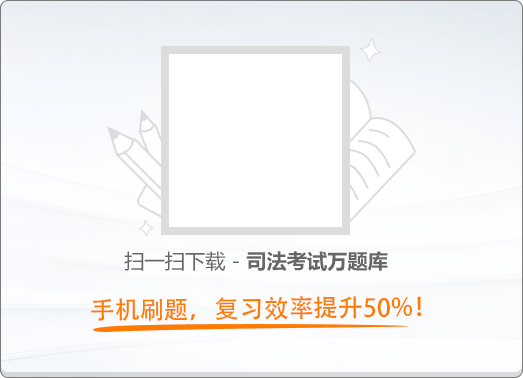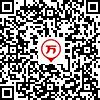180:002014年《国家司法考试（卷三）》真题

1
(单项选择题)

• A.

薛某是义务人，但无对应权利人，让薛某承担赔偿义务，违反了权利义务相一致的原则

• B.

交警大队未受损失而保有6万元，形成不当得利，应予退还

• C.

交警大队代收6万元，依法行使行政职权，与薛某形成合法有效的行政法律关系，无须退还

• D.

如确实未找到权利人，交警大队代收的6万元为无主财产，应收归国库

• A
• B
• C
• D

2
(单项选择题)

• A.

李某、张某不得解除收养关系

• B.

李某应对张某承担违约责任

• C.

张某应赔偿李某1万元

• D.

李某应返还不当得利

• A
• B
• C
• D

3
(单项选择题)

• A.

第四条为无效格式条款

• B.

乙公司法定代表人未在第四条处签字

• C.

乙公司法定代表人的签字仅代表乙公司的行为

• D.

李蓝并未在合同上签字

• A
• B
• C
• D

4
(单项选择题)

• A.

应归甲公司所有

• B.

应归宗某继承人所有

• C.

应按比例退还员工

• D.

应用于同类公益事业

• A
• B
• C
• D

5
(单项选择题)

• A.

乙公司的书面答复意味着乙公司需偿还甲公司3万元

• B.

乙公司的书面答复构成要约

• C.

乙公司的书面回函对甲公司有效

• D.

乙公司的书面答复表明其丧失了10万元的时效利益

• A
• B
• C
• D

6
(单项选择题)

• A.

李某构成违约，张某可请求李某返还5万元

• B.

李某构成违约，张某可请求李某返还6万元

• C.

李某构成侵权，张某可请求李某返还5万元

• D.

李某构成侵权，张某可请求李某返还6万元

• A
• B
• C
• D

7
(单项选择题)

• A.

10万元

• B.

9万元

• C.

8万元

• D.

7万元

• A
• B
• C
• D

8
(单项选择题)

• A.

乙公司应先行使机器设备抵押权

• B.

乙公司应先行使房屋抵押权

• C.

乙公司应先行请求丙公司承担保证责任

• D.

丙公司和丁公司可相互追偿

• A
• B
• C
• D

9
(单项选择题)

• A.

张某拾得小羊后因占有而取得所有权

• B.

张某有权要求王某返还占有

• C.

张某有权要求李某返还占有

• D.

李某侵犯了张某的占有

• A
• B
• C
• D

10
(单项选择题)

• A.

丙公司保证担保的主债权为300万元

• B.

丙公司保证担保的主债权为500万元

• C.

丙公司保证担保的主债权为600万元

• D.

因延长还款期限未经保证人同意，丙公司不再承担保证责任

• A
• B
• C
• D

11
(单项选择题)

• A.

汤某有权要求余某承担违约责任

• B.

汤某有权要求朱某承担侵权责任

• C.

方某有权要求朱某承担侵权责任

• D.

方某有权要求余某承担违约责任

• A
• B
• C
• D

12
(单项选择题)

• A.

甲公司不构成违约

• B.

乙公司有权解除合同

• C.

乙公司可行使先履行抗辩权

• D.

乙公司可要求提高合同价格

• A
• B
• C
• D

13
(单项选择题)

• A.

因2006年的借款已到期，故归还的是该笔借款

• B.

因2006年的借款无担保，故归还的是该笔借款

• C.

因2006年和2009年的借款数额相同，故按比例归还该两笔借款

• D.

因2006年和2009年的借款均有担保，故按比例归还该两笔借款

• A
• B
• C
• D

14
(单项选择题)

• A.

李某有权要求王某搬离房屋

• B.

陈某有权要求王某搬离房屋

• C.

李某有权解除合同，要求孙某承担赔偿责任

• D.

陈某有权解除合同，要求孙某承担赔偿责任

• A
• B
• C
• D

15
(单项选择题)

• A.

李某与甲银行关于直接在账户内扣划款项的约定无效

• B.

李某无须承担保证责任

• C.

乙支行收回20万元全部借款本金和利息之前，李某不得向张某追偿

• D.

乙支行应以自己的名义向张某行使追索权

• A
• B
• C
• D

16
(单项选择题)

• A.

乙公司依法获得药品生产许可证之前，专利申请权转让合同未生效

• B.

专利申请权的转让合同自向国务院专利行政主管部门登记之日起生效

• C.

专利申请权的转让自向国务院专利行政主管部门登记之日起生效

• D.

如该专利申请因缺乏新颖性被驳回，乙公司可以不能实现合同目的为由请求解除专利申请权转让合同

• A
• B
• C
• D

17
(单项选择题)

• A.

甲展览馆许可乙博物馆异地重建完全相同的雕塑

• B.

甲展览馆仿照雕塑制作小型纪念品向游客出售

• C.

个体户冯某仿照雕塑制作小型纪念品向游客出售

• D.

游客陈某未经著作权人同意对雕塑拍照纪念

• A
• B
• C
• D

18
(单项选择题)

• A.

侵犯了主办方对篮球比赛的著作权

• B.

侵犯了篮球运动员的表演者权

• C.

侵犯了舞蹈演员的表演者权

• D.

侵犯了主办方的广播组织权

• A
• B
• C
• D

19
(单项选择题)

• A.

乙公司的行为属于仿冒注册商标

• B.

丙公司可继续销售“山叶”小轿车

• C.

丙公司应赔偿甲公司损失100万元

• D.

工商行政管理部门不能对丙公司进行罚款处罚

• A
• B
• C
• D

20
(单项选择题)

• A.

甲主观上为避免自家房屋受损，不构成无因管理，应自行承担医疗费用

• B.

甲依据无因管理只能向乙主张医疗费赔偿，因乙是房屋所有人

• C.

甲依据无因管理只能向丙主张医疗费赔偿，因丙是房屋实际使用人

• D.

甲依据无因管理不能向A公司主张医疗费赔偿，因甲欠缺为A公司的利益实施管理的主观意思

• A
• B
• C
• D

21
(单项选择题)

• A.

对王某的赔偿责任应由李某承担，黄某承担补充责任

• B.

对王某的赔偿责任应由甲公司承担，乙公司承担补充责任

• C.

甲公司与乙公司应对王某承担连带赔偿责任

• D.

对王某的赔偿责任承担应采用过错责任原则

• A
• B
• C
• D

22
(单项选择题)

• A.

欣欣医院构成违约行为和侵权行为

• B.

欢欢的继承人可继承欣欣医院对欢欢支付的精神损害赔偿金

• C.

洋洋的行为侵犯了欢欢的名誉权

• D.

欢欢的母亲可以欢欢的名义对洋洋提起侵权之诉

• A
• B
• C
• D

23
(单项选择题)

• A.

甲与乙离婚时，乙无权请求将门面房作为夫妻共同财产分割

• B.

甲与丁的协议应视为双方约定处分共同财产

• C.

如甲、丁离婚，有关医疗费按借款协议约定处理

• D.

如丁不同意甲支付医疗费，甲无权要求分割共有财产

• A
• B
• C
• D

24
(单项选择题)

• A.

• B.

• C.

• D.

乙、丙、丁

• A
• B
• C
• D

25
(单项选择题)

• A.

在北京市设立分公司，不必申领分公司营业执照

• B.

在北京市以外设立分公司，须经登记并领取营业执照，且须独立承担民事责任

• C.

在北京市以外设立分公司，其负责人只能由张某、刘某、姜某、方某中的一人担任

• D.

在北京市以外设立子公司，即使是全资子公司，亦须独立承担民事责任

• A
• B
• C
• D

26
(单项选择题)

• A.

在公司章程未特别规定时，甲、乙的继承人均可主张股东资格继承

• B.

在公司章程未特别规定时，甲的继承人可以主张继承股东资格与董事长职位

• C.

公司章程可以规定甲、乙的继承人继承股东资格的条件

• D.

公司章程可以规定甲、乙的继承人不得继承股东资格

• A
• B
• C
• D

27
(单项选择题)

• A.

在严某认缴公司章程所规定的出资后，公司即须签发出资证明书

• B.

若严某遗失出资证明书，其股东资格并不因此丧失

• C.

出资证明书须载明严某以及其他股东的姓名、各自所缴纳的出资额

• D.

出资证明书在法律性质上属于有价证券

• A
• B
• C
• D

28
(单项选择题)

• A.

可同时提起清算公司的诉讼

• B.

可向法院申请财产保全

• C.

可将其他股东列为共同被告

• D.

如法院就解散公司诉讼作出判决，仅对公司具有法律拘束力

• A
• B
• C
• D

29
(单项选择题)

2014年5月，甲、乙、丙三人共同出资设立一家有限责任公司。甲的下列哪一行为不属于抽逃出资行为？（　）

• A.

将出资款项转入公司账户验资后又转出去

• B.

虚构债权债务关系将其出资转出去

• C.

利用关联交易将其出资转出去

• D.

制作虚假财务会计报表虚增利润进行分配

• A
• B
• C
• D

30
(单项选择题)

2010年5月，贾某以一套房屋作为投资，与几位朋友设立一家普通合伙企业，从事软件开发。2014年6月，贾某举家移民海外，故打算自合伙企业中退出。对此，下列哪一选项是正确的？（　）

• A.

在合伙协议未约定合伙期限时，贾某向其他合伙人发出退伙通知后，即发生退伙效力

• B.

因贾某的退伙，合伙企业须进行清算

• C.

退伙后贾某可向合伙企业要求返还该房屋

• D.

贾某对退伙前合伙企业的债务仍须承担无限连带责任

• A
• B
• C
• D

31
(单项选择题)

2014年6月经法院受理，甲公司进入破产程序。现查明，甲公司所占有的一台精密仪器，实为乙公司委托甲公司承运而交付给甲公司的。关于乙公司的取回权，下列哪一表述是错误的？（　）

• A.

取回权的行使，应在破产财产变价方案或和解协议、重整计划草案提交债权人会议表决之前

• B.

乙公司未在规定期限内行使取回权，则其取回权即归于消灭

• C.

管理人否认乙公司的取回权时，乙公司可以诉讼方式主张其权利

• D.

乙公司未支付相关运输、保管等费用时，保管人可拒绝其取回该仪器

• A
• B
• C
• D

32
(单项选择题)

• A.

没有票据，就没有票据权利

• B.

任何类型的票据都必须能够进行转让

• C.

票据的效力不受票据赖以发生的原因行为的影响

• D.

票据行为的方式若存在瑕疵，不影响票据的效力

• A
• B
• C
• D

33
(单项选择题)

• A.

船舶买卖时，船舶所有权自船舶交付给买受人时移转

• B.

船舶建造完成后，须办理船舶所有权的登记才能确定其所有权的归属

• C.

船舶不能成为共同共有的客体

• D.

船舶所有权不能由自然人继承

• A
• B
• C
• D

34
(单项选择题)

• A.

谢某代签字，应由谢某承担保险责任

• B.

甲公司承保错误，无须承担保险责任

• C.

何某已经交纳了保险费，应由甲公司承担保险责任

• D.

何某默认谢某代签字有过错，应由何某和甲公司按过错比例承担责任

• A
• B
• C
• D

35
(单项选择题)

• A.

检察监督原则

• B.

诚实信用原则

• C.

当事人诉讼权利平等原则

• D.

同等原则和对等原则

• A
• B
• C
• D

36
(单项选择题)

• A.

法院主动对确有错误的生效调解书启动再审

• B.

派出所民警对民事纠纷进行调解

• C.

法院为下落不明的被告指定代理人参加调解

• D.

人民调解委员会主动调解当事人之间的民间纠纷

• A
• B
• C
• D

37
(单项选择题)

• A.

当事人以欺骗的方法形成不正当诉讼状态

• B.

证人故意提供虚假证言

• C.

法院根据案件审理情况对当事人提供的证据不予采信

• D.

法院对当事人提出的证据任意进行取舍或否定

• A
• B
• C
• D

38
(单项选择题)

• A.

被告以袁某是原告的近亲属为由申请其回避，法院应批准

• B.

袁某在庭上的陈述是一种法定证据

• C.

被告可对袁某进行询问

• D.

袁某出庭的费用，由败诉方当事人承担

• A
• B
• C
• D

39
(单项选择题)

• A.

军人与非军人之间的民事诉讼，都应由军事法院管辖，体现了专门管辖的原则

• B.

中外合资企业与外国公司之间的合同纠纷，应由中国法院管辖，体现了维护司法主权的原则

• C.

最高法院通过司法解释授予部分基层法院专利纠纷案件初审管辖权，体现了平衡法院案件负担的原则

• D.

不动产纠纷由不动产所在地法院管辖，体现了管辖恒定的原则

• A
• B
• C
• D

40
(单项选择题)

• A.

应在开庭审理时先行调解

• B.

应开庭审理，但经过赵洪和陈海的书面同意后，可书面审理

• C.

应当庭宣判

• D.

应一审终审

• A
• B
• C
• D

41
(单项选择题)

• A.

法院受理第三人撤销之诉后，应中止原裁判的执行

• B.

第三人撤销之诉是确认原审裁判错误的确认之诉

• C.

第三人撤销之诉由原审法院的上一级法院管辖，但当事人一方人数众多或者双方当事人为公民的案件，应由原审法院管辖

• D.

第三人撤销之诉的客体包括生效的民事判决、裁定和调解书

• A
• B
• C
• D

42
(单项选择题)

• A.

向张某送达举证通知书

• B.

向张某送达缺席判决书

• C.

向海斯送达举证通知书

• D.

向海斯送达缺席判决书

• A
• B
• C
• D

43
(单项选择题)

• A.

刘某的支付租金请求权已经超过诉讼时效

• B.

租赁合同无效

• C.

自己无支付能力

• D.

自己已经支付了租金

• A
• B
• C
• D

44
(单项选择题)

• A.

由于本案标的金额巨大，且具有涉外因素，银行应向W市中院提交书面申请

• B.

本案的被申请人只应是债务人甲公司

• C.

如果法院经过审查，作出拍卖裁定，可直接移交执行庭进行拍卖

• D.

如果法院经过审查，驳回银行申请，银行可就该抵押权益向法院起诉

• A
• B
• C
• D

45
(单项选择题)

• A.

经过公证的书证，其证明力一般大于传来证据和间接证据

• B.

经验法则可验证的事实都不需要当事人证明

• C.

在法国居住的雷诺委托赵律师代理在我国的民事诉讼，其授权委托书需要经法国公证机关证明，并经我国驻法国使领馆认证后，方发生效力

• D.

证明责任是一种不利的后果，会随着诉讼的进行，在当事人之间来回移转

• A
• B
• C
• D

46
(单项选择题)

• A.

支付令不能留置送达，法官的送达无效

• B.

提出支付令异议的期间是10天，陈某的异议不发生效力

• C.

陈某的异议并未否认二人之间存在借贷法律关系，因而不影响支付令的效力

• D.

法院应将本案转为诉讼程序审理

• A
• B
• C
• D

47
(单项选择题)

• A.

由于原判已被撤销，一审中的审判行为无效，保全措施也应解除

• B.

由于原判已被撤销，一审中的诉讼行为无效，法院必须重新指定举证时限

• C.

重审时不能再适用简易程序，应组成合议庭，李法官可作为合议庭成员参加重审

• D.

若重审法院判决甲胜诉，乙再次上诉，二审法院认为重审认定的事实依然错误，则只能在查清事实后改判

• A
• B
• C
• D

48
(单项选择题)

• A.

书证

• B.

鉴定意见

• C.

勘验笔录

• D.

电子数据

• A
• B
• C
• D

49
(单项选择题)

• A.

向执行法院申请复议

• B.

向执行法院的上一级法院申请复议

• C.

向执行法院提起异议之诉

• D.

向原审法院申请再审

• A
• B
• C
• D

50
(单项选择题)

• A.

裁定撤诉，视为撤回起诉

• B.

裁定撤诉，视为撤回再审申请

• C.

裁定诉讼中止

• D.

缺席判决

• A
• B
• C
• D